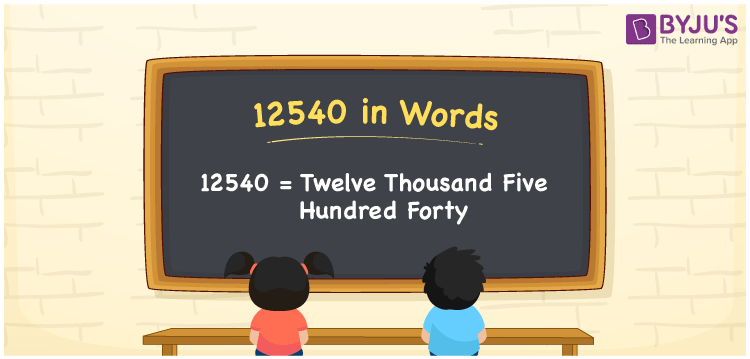# 12540 in Words

12540 in words is “Twelve thousand five hundred forty”. The natural number 12540 lies between 12539 and 12541. It is also considered to be a cardinal number. For example, there are 12540 students studying in a school. This article will help you to learn the number 12540 in words and its procedure in detail.

 12540 in Words: Twelve Thousand Five Hundred Forty. Twelve Thousand Five Hundred Forty in Numerical Form: 12540.

## 12540 in English Words## How to Write 12540 in Words?

Following is the place value table for the number 12540.

 Ten-thousands Thousands Hundreds Tens Ones 1 2 5 4 0

The expanded form of 12540 is as follows:

= 1 × Ten thousand + 2 × Thousand + 5 × Hundred + 4 × Ten + 0 × One

= 1 × 10000 + 2 × 1000 + 5 × 100 + 4 × 10 + 0 × 1

= 10000 + 2000 + 500 + 40

= 12540

= Twelve thousand five hundred forty

Hence, 12540 in words is twelve thousand five hundred forty.

12540 in words – Twelve thousand five hundred forty

Is 12540 an odd number? – No

Is 12540 an even number? – Yes

Is 12540 a perfect square number? – No

Is 12540 a perfect cube number? – No

Is 12540 a prime number? – No

Is 12540 a composite number? – Yes

## Frequently Asked Questions on 12540 in Words

Q1

### How to write 12540 in English words?

12540 in words is twelve thousand five hundred forty.

Q2

### Simplify 12000 + 540, and express it in words.

Simplifying 12000 + 540, we get 12540. Hence, 12540 in words is twelve thousand five hundred forty.

Q3

### Is 12540 a composite number?

Yes, 12540 is a composite number.